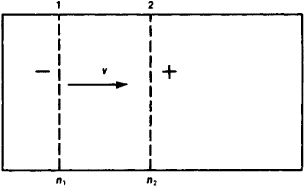# ambipolar diffusion

Also found in: Wikipedia.

## ambipolar diffusion

(am-bee-poh -ler) A process by which magnetic flux and angular momentum are removed from dense cores in molecular clouds, ultimately leading to the collapse of the core and the formation of a low-mass star. See star formation.

## Ambipolar Diffusion

or bipolar diffusion, the simultaneous transport, in an ionized medium, of charged particles of both signs (for example, electrons and positive ions), leading to a decrease of their concentration in the medium. Ambipolar diffusion can be seen very clearly in gas and liquid plasmas, which are ionized mediums where the densities of charge of both signs are equal to each other. If in such a medium the concentrations of charge carriers n+ and n~ change from one point to another (for example, decrease from level 1 to level 2, as shown in Figure 1), then the charge carriers (ions or electrons) diffuse from 1 to 2. The charge carriers which diffuse faster (for example, the positive ones) will at first move in great numbers from 1 to 2. As a result, level 2 will have an excess of that charge (in this case, positive) and level 1 will have an excess of the opposite charge. Therefore, an electric field will form between levels 1 and 2 (in this case, directed from 2 to 1); this will slow the movement of the more mobile carriers and speed the movement of the slower carriers. An equilibrium state will be reached when the intensity of field E is such that the transference velocities of charge carriers of both signs are equal to each other. This is what characterizes ambipolar diffusion. The flux of charge carriers v of either sign is determined by the combined effect of diffusion and the electrical field. The velocity of ambipolar diffusion is governed by a law similar to that governing the velocity of ordinary diffusion, but with a different coefficient.Figure 1

Ambipolar diffusion takes place in liquids (electrolytes) when there is a difference in electrolyte concentrations. For example, when an acid is poured into water, the cations H+, which are more mobile than the acid anions, overtake the latter and produce a positive potential in regions of lower concentration. The resultant difference in potential between regions of different concentration can be measured and serves as a measure of the concentrational electromotive force which appears as a result of ambipolar diffusion.

Ambipolar diffusion can also take place in semiconductors which have free carriers of charges of both signs. Ambipolar diffusion plays an important role in gas discharge devices (phanotrons, thyratrons, and others).

## ambipolar diffusion

[¦am·bē′pōl·ər dif′yü·zhən]
(physics)
The diffusion in a plasma of charged particles, such as electrons or ions, as a result of the almost exact local charge neutrality required.
Mentioned in ?
References in periodicals archive ?
where [[tau].sub.e] is the effective carrier lifetime, [alpha] the absorption coefficient of the semiconductor at the illumination wavelength, [P.sub.0] the light intensity, [omega] the angular frequency of the light, [??] the reduced Planck constant, R the reflectivity of the wafer, and Ld the ambipolar diffusion length calculated using the diffusion characteristics of both electrons and holes .
The free carriers that diffuse out of the illuminated areas both lower the photogenerated carrier concentration as well as expand the effective size of the photoconductive patterns, with a size scale comparable to the ambipolar diffusion length.
Description Value [alpha] Absorption coefficient at 550 5.03 x [10.sup.5] nm optical illumination [cm.sup.-1] [D.sub.a] Ambipolar diffusion coefficient 63.88 [cm.sup.2]/s [[tau].sub.e] Effective carrier lifetime 40 x [10.sup.-6]s [L.sub.D] Ambipolar diffusion length 5.05 x [10.sup.-2] cm [[mu].sub.e] Electron mobility 3900 [cm.sup.2]/Vs [[mu].sub.h] Hole mobility f900 [cm.sup.2]/Vs
This phenomenon, proposed by Schottky , is called ambipolar diffusion [72, 73].
However, the negative charges on the surface will immediately be balanced by an equal number of oppositely charged [Si.sup.+] and [O.sup.+] ions because these positive ions move together with the electrons as a result of ambipolar diffusion. In this way, an atmosphere of ions is formed in the rapid thermal motion close to the surface.
Rose, "The transition from free to ambipolar diffusion," Physical Review, vol.
For instance, ambipolar diffusion is predicted to dominate in the tenuous hot corona of the disc.
If the electron and hole densities are assumed to be equal, then 2/D = 1/[D.sub.n] + 1/[D.sub.p], which means that the ambipolar diffusion current is the sum of electrons and holes.
One of the accomplishments has involved gaining an understanding of what is known as ambipolar diffusion, in which both the electrons and the positively charged ions in protoplanetary disks are dragged along by a magnetic field.
Simon explained that so far the most important outcome of his team's research into protoplanetary disk turbulence is the discovery that for turbulence levels to be large enough to agree with what observations suggest, a magnetic field perpendicular to the disk must be present; otherwise, the turbulence is rather weak because the ambipolar diffusion quenches the turbulence.
He said that the perpendicular magnetic field creates more vigorous turbulence that can overpower the ambipolar diffusion in some regions.

Site: Follow: Share:
Open / Close